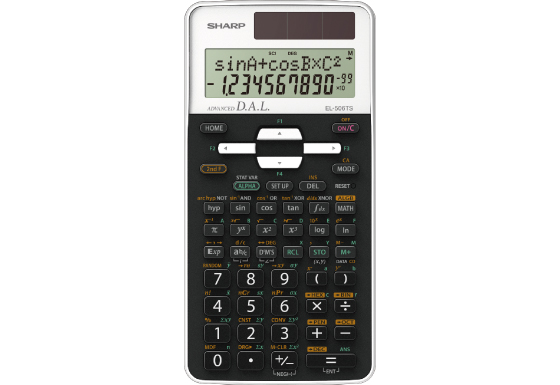## EL-506TS

The advanced scientific model EL-506TS performs 470 statistical, trigonometric and mathematical functions including metric conversions and physical constants, making it suitable for high school and university students. Large 2-line 10+2 digits display allows browsing and editing of previous calculations, and hard cover to protect calculator from scratches and falls.Specification

• Built-In Functions: 470
• Entry System: Algebraic (D.A.L.)
• Power Source: Solar & Battery
• Dimensions and Weight: 161 × 80 × 15 mm, 110 g

Mathematical Functions

• Fractions
• Equation Solver (Newton)
• System of Linear Equations
• Matrix and Vector Calculations
• Numeric Derivative and Integral
• Complex Number Calculation

Notation and Decimal Places

• FSE Display (SCI, FIX, ENG)
• 3-Digit Punctuation

Statistics

• 1-Variable Statistics
• 2-Variable Statistics
• Regression Calculations: 6
• Mean, Standard Deviation
• nCr, nPr, n!
• Random Numbers

Memory

• Multi-Line Playback
• Memory Variables: 9

Trigonometric Functions

• Sin, Cos, Tan and Inverses
• Hyperbolic Functions
• Sexagesimal and Decimal Conversions
• Rectangular and Polar Coordinates Conversions

Number Systems

• Number Systems: DEC, BIN, OCT, HEX, PEN
• Logical Operation (AND, OR, NOT, NEG, XOR)

Scientific Functions

• Metric Conversions
• Physical Constants